﻿ On the Numerical Regularity in the aspect of Prime Numbers

### On the Numerical Regularity in the aspect of Prime NumbersOPEN ACCESSPEER-REVIEWED

## On the Numerical Regularity in the aspect of Prime Numbers

Mathematical Society, Foundation Public School, Karachi, Pakistan

### Abstract

The purpose of this paper is to introduce a new pattern in Primes numbers, to eliminate the randomness in their patterns. This paper also justifies the solutions in a numerical and geometric manner. The Prime Function provides further distinction in the nature of Prime Numbers by distinguishing the nature of normality and Abnormality in Prime Numbers. To verify the normality of corresponding Prime numbers, the Sufi primality test is formed. Also using the Prime Function, the formula for the approximate sum of Prime Numbers is derived. The limitations and conditions of the Prime function are also stated. These factors provide a panoramic view of the Prime Function and its potential factor in Number Theory .

• Sufi, Shaad P.. "On the Numerical Regularity in the aspect of Prime Numbers." Turkish Journal of Analysis and Number Theory 3.1 (2015): 12-16.
• Sufi, S. P. (2015). On the Numerical Regularity in the aspect of Prime Numbers. Turkish Journal of Analysis and Number Theory, 3(1), 12-16.
• Sufi, Shaad P.. "On the Numerical Regularity in the aspect of Prime Numbers." Turkish Journal of Analysis and Number Theory 3, no. 1 (2015): 12-16.

 Import into BibTeX Import into EndNote Import into RefMan Import into RefWorks

### 1. Introduction

Like the geometrical interpretation of the pattern in Prime Numbers in Ulam Spiral, there does exist arithmetic interpretation of Prime Numbers. The representation of the arithmetic interpretation is presented by the following function(1)

It had been long known that the pattern of occurrence of prime numbers follow the Randomness Principle presented in Chaos Theory. However, the Ulam spiral has provided a geometric interpretation to falsify the previous statement. This paper provides a deterministic approach to generalize the pattern that exists between Prime numbers. The Prime Function is an arithmetic representation of Prime Numbers. This Function provides approximate solutions for the variablewhich is in a range of 2 units of the corresponding Prime Number. The corresponding Prime Number to the solution of the Prime Function (see Sec. 1) which lies beyond this specified range is considered as an Abnormal Prime Number (see Sec.3). The Prime Function also provides an approximation for the sum of all Prime Numbers up till PN, which is the largest Prime numbers

Where it is considered that liminfn→∞(pn+1−pn) <7×107, Where pn is the nth prime .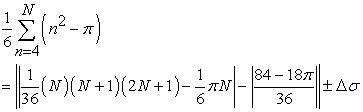The Prime function also provides the basis of the Sufi Primality Test (see Sec. 5). In this function, the Sufi test is a primality test for a variable; it requires the definite integral of the Prime function be already known Where the upper limit of the definite integral is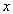.

It is the basis of the Prime Function that gives a concise verification that the corresponding Prime number to the solution of the functionis a Normal Prime (see Sec.3).

### 2. The Limitations and Conditions of the Prime Function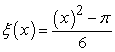The generalization of the arithmetic approach to Prime numbers is dependent upon certain conditions of the Prime Function. These limiations of the function are applied to the variable, the limits of the irrational solution and the range of qualification of the solution for the distinction between Normal and Abnormal primes (see Sec.3).

The variable, presented in the Prime Function, is bounded to be an real positive integer. Also, this variable should satisfy the following equation whereWhere= 4 is associated to the smallest corresponding Prime number, P1, of the sequence.

The arthemetic sequence mentioned in Table 1 relates the variable of the Prime function and the corresponding Prime Number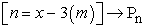Where Pn is the nth prime number,

And m is the number of term.

The inclusive, irrational constant,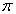, in the Prime function limits the function to contain an integer solution. Therefore to simplify and compare the indifference between the Prime Function and the Prime Numbers, the lower limit of the solution of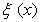is taken.

#### Table 2. This table presents that the lower limit of the solution of the Prime Function is taken to simplify and compare the indifference between the Prime Function and the Prime Numbers

Furthermore, the validity of the lower limit solution terminates when the initial two decimal places of the solution ofare equal to 97Where De = the initial two decimal places of a solution of the Prime Function. Prepresents the solution of the corresponding Normal Prime. If this is the case then the upper limit of the solution ofis considered.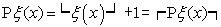#### Table 3. This table presents that the upper limit of the solution of ξ(x) is considered when De=97

The distinction between the Normal and Abnormal Primes is based on the phenomenon of range of solution in the Prime Function. The solution of the Prime Functionfor the variabletaken to the lower limit, or upper limit when De= 97, should be within the range of two units for its corresponding Prime number Pn to be considered Normal Prime. If this condition is not satisfied, the corresponding Prime number Pn of the variableis considered as an Abnormal Prime. The range of the solution ofis as follows :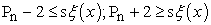Where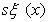represents the solution of the Prime Function

### 3. Interpretations of the Graphical Representation of Prime Function

The indifference between the solutions of the Prime Function and the Prime Number Sequnce is represented graphically. This representation confirms the accuracy of the Prime Function.

The Continuity Function averagely follows the natural sequence of Prime numbers in the range of two units from the natural positive patterns of the prime numbers. The graph shows the solutions of the Continuity Function against the natural sequence of the Prime Numbers. Slight fluctuations may be seen between the both functions. These graphical fluctuations represent the maximum differecnce between the solution of the Prime Function and the Prime Number Sequence. The variable M in the function is arranged linearly according to the function

Where the condition≥4 is established. The solutions of the function for≥4 is graphed accordingly to the prime numbers due to the conditions of The Continuity Function.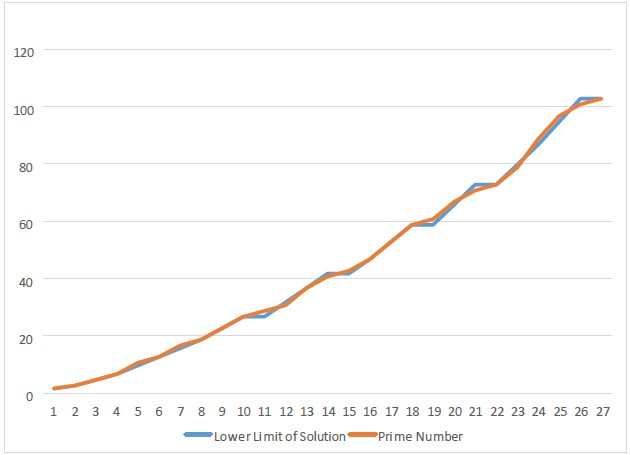### 4. Abnormal PrimesThe Prime Function has caused yet another differentiation in the nature of Prime Numbers. This differentiation distinguishes Prime Numbers between their nature of Normality and Abnormality

To perceive an Abnormal Prime, one has to recognize the limits of the range of solutions of the Prime Function (see Sec.1). The condition states that an Abnormal Prime is a real prime number which does not satisfy the condition of Normal Primes. In order for a Prime Number to be considered Normal, its corresponding solution of the Prime Function should be within the range of 2 units from it.i.e. The maximum difference between the solution of the Prime Function and the corresponding Prime number should be of 2 units.

The condition of the Abnormal prime isAbnormal Primes are taken into consideration as a sufficient number of corresponding Prime numbers satisfy conditions of the Normal Primes. Therefore corresponding prime numbers which satisfy Abnormal Prime condition are listed as a different set. (See Table 4)A= Set of Normal Prime Numbers

B= Set of Abnormal Prime Numbers.

A prime number may not exist as both Normal and Abnormal Prime.

Numerical Representation of the Solutions of the Prime Function

#### Table 4. This table shows that a sufficient number of corresponding Prime numbers satisfy conditions of the Normal Primes as ∆= 0; where M= x

In Table 4, 27 solutions of the Prime Function are considered. All Primes numbers of the corresponding solutions satisfy the condition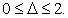This shows that all corresponding Prime numbers are Normal in nature and the probability of encountering a Abnormal Prime is extremely low, in consideration of uncertainty.

### 5. Summation of the Prime Number Series by Prime Function

It is now known that the solutions of the Prime Function reproduce their corresponding Prime Number to a corresponding accuracy (see Table 4). Therefore it may be subjected that the summation of the Prime Function may approximate the sum of Prime Numbers for values ofwhere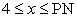Where PN is the largest Prime Number.

The summation of particular Prime Numbers includes variable uncertainty due to the consideration of upper and lower limits of solutions of the Prime Function. Also the solution of this summation involves both Normal and Abnormal Prime Numbers regardless of their nature.Since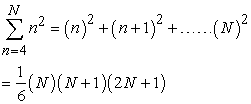Where N is a variable of the Prime Function and thereforeOne may notice that the summation function has a lower limit of n=4. Therefore the lower limit of the summation is changed to n=0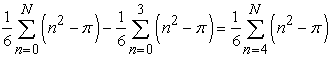The coefficient terms in the expansion are then converted into arbitrary constants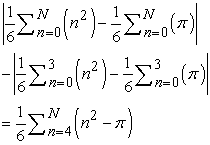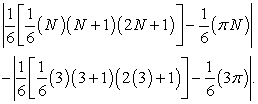To simplify the above expression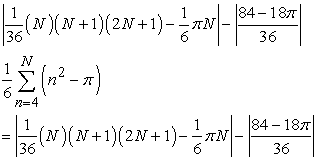The above expression indicates the summation of Prime numbers up till N. This expression, however, lacks the indication of the absolute uncertainty of the summation. Therefore to complete the expression,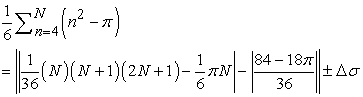Since the upper and lower limit of the solutions of the Prime Function cause a maximum difference of 1 unit between the calculated and rounded solutions, N=1 is taken as the uncertainty of calculation.The absolute uncertainty causes the summation of the Prime numbers for a specific range.

Therefore the final Summation Equation for the Summation of Prime Numbers is as follows### 6. Sufi Primality Test of Normal Primes

The confirmation of distinction between Normal and Abnormal Primes is an essential objective of this paper. Therefore to assure the interpretation of the Sufi Primality Test is of essential importance. The Sufi Primality test provides further confirmation about a corresponding Prime number of a solution of Prime Function through the integration of the Prime Function. The Sufi test is a primality test for a variable; it requires the definite integral of the Prime function be already known (3)

Where the upper limit of the definite integral is.It is the basis of the Prime Function that gives a concise verification that the corresponding Prime number to the solution of the functionis a Normal Prime.

This Test states that if the solution of the Prime Function lies within a 2 unit range of the corresponding Prime number then the result of the definite integral of upper limitand lower limit of 0 will also lie within a 2 unit range of that corresponding Prime Number. This confirms that the corresponding Prime number of variableis a Normal prime number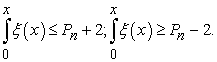Since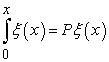The integral of the function is as follows: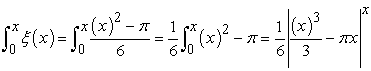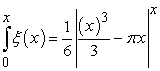Hence if### References

  https://www.maa.org/sites/default/files/pdf/upload_library/22/Ford /Granville.pdf.In article  http://annals.math.princeton.edu/2014/179-3/p07.In article  http://en.wikipedia.org/wiki/Lucas_primality_test.In article